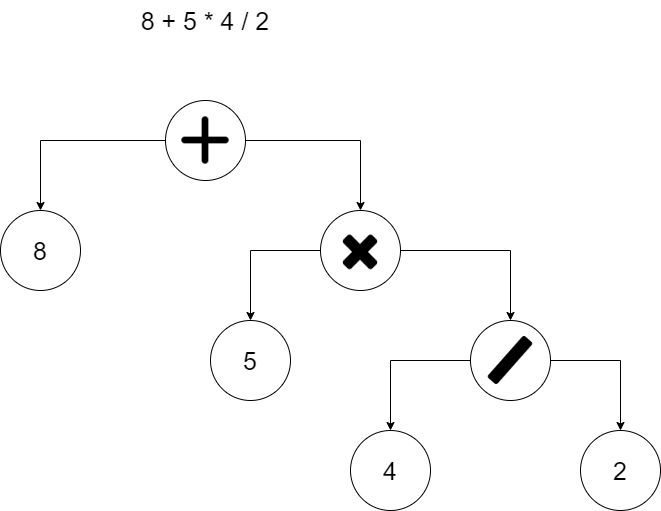How To Make A Language: Parsing

The last tutorial didn't really give an in depth explanation as to what everything was doing aside from some comments, however for parsing I feel I should explain how it works.

Markdown was really weird on this post.

We are going to be using a recursive descent parser, written all by hand and with no dependencies.

Lets start with an expression `8 + 5 * 4 / 2`

The first thing we are going to do is tokenize it, so this expression will tokenize to this

``````1: ("Number", 8)
2: ("Operator", "+")
3: ("Number", 5)
4: ("Operator", "*")
5: ("Number", 4)
6: ("Operator", "/")
7: ("Number", 2)``````

Im going to do a little experiment here, and if it works you should be able to read the above stream of tokens just like an rdp parser. When you see `Add(), Sub(), Mul(), Div() and Literal()` in the text below jump to the corresponding label!

Left = Sub()

``````Is the next token a + operator? If so then:
Right = Sub()
Return (Left, "+", Right)

Otherwise return the left side``````

Sub:
Left = Mul()

``````Is the next token a - operator? If so then:
Right = Mul()
Return (Left, "-", Right)

Otherwise return the left side``````

Mul:
Left = Div()

``````Is the next token a * operator? If so then:
Right = Div()
Return (Left, "*", Right)

Otherwise return the left side``````

Div:
Left = Literal()

``````Is the next token a / operator? If so then:
Right = Literal()
Return (Left, "/", Right)

Otherwise return the left side``````

Literal:
Return the current token and advance to the next one

Now, if you went through everything above and kept track of where you were in the sequence you will notice that you end with a tree that looks roughly like this:You are viewing a single comment. View All
LoganSpong (52)

@BXRStudios No, I'm the 10 year old that made the uhh... thing.